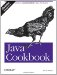# Recipe 6.7 Adding to or Subtracting from a Dateor Calendar

#### Problem

You need to add or subtract a fixed amount to or from a date.

#### Solution

As we've seen, Date has a getTime( ) method that returns the number of seconds since the epoch as a long . To add or subtract, you just do arithmetic on this value. Here's a code example:

`// DateAdd.java  /** Today's date */  Date now = new Date( );    long t = now.getTime( );    t -= 700L*24*60*60*1000;    Date then = new Date(t);    System.out.println("Seven hundred days ago was " + then);`

#### Discussion

A cleaner variant is to use the Calendar's add( ) method. There is no corresponding subtraction method; you just add a negative value to make time run backward:

`import java.text.*; import java.util.*; /** DateCalAdd -- compute the difference between two dates.  */ public class DateCalAdd {     public static void main(String[] av) {         /** Today's date */         Calendar now = Calendar.getInstance( );         /* Do "DateFormat" using "simple" format. */         SimpleDateFormat formatter             = new SimpleDateFormat ("E yyyy/MM/dd 'at' hh:mm:ss a zzz");         System.out.println("It is now " +              formatter.format(now.getTime( )));         now.add(Calendar.YEAR, - 2);         System.out.println("Two years ago was " +              formatter.format(now.getTime( )));     } }`

Running this reports the current date and time, and the date and time two years ago:

`> java DateCalAdd It is now Tue 2003/11/25 at 09:14:26 AM EST Two years ago was Sun 2001/11/25 at 09:14:26 AM EST`

A method called roll( ) does not change "larger" fields. For example, rolling the month up from January 31, 2051 results in February 28, 2051.Java Cookbook, Second Edition
ISBN: 0596007019
EAN: 2147483647
Year: 2003
Pages: 409
Authors: Ian F Darwin

Similar book on Amazon